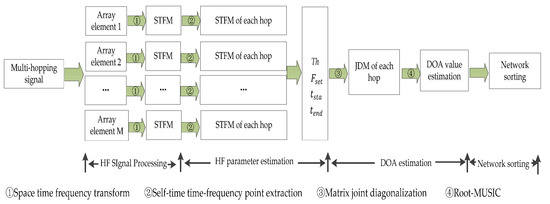Article

# Parameter Estimation of Multi Frequency Hopping Signals Based on Space-Time-Frequency Distribution

College of Information and Communication Engineering, Harbin Engineering University, Harbin 150001, China
*
Author to whom correspondence should be addressed.
Symmetry 2019, 11(5), 648; https://doi.org/10.3390/sym11050648
Received: 13 April 2019 / Revised: 30 April 2019 / Accepted: 5 May 2019 / Published: 8 May 2019
Frequency hopping spread spectrum (FHSS) communication is widely used in military and civil communication, and the parameter estimation of frequency hopping (HF) signals is of great significance. In order to estimate the parameters of multiple frequency hopping signals effectively, a blind parameter estimation algorithm based on space-time frequency analysis (STFA) and matrix joint diagonalization (JDM) is proposed. Firstly, the time domain signal received by the linear array is converted to the space-time frequency domain through the space-time frequency transformation, and the space-time frequency distribution (STFD) of the signal is obtained. Then the time-frequency point is extracted from the space-time frequency distribution map, the extraction of the hop is completed by the method of finding an “island”, and the space-time frequency matrix of each hop is constructed, and then the preliminary estimation of each jump frequency, jump time and jump period is completed. Finally, the space-time-frequency matrix of the same hop received by different array elements is jointly diagonalized by the matrix joint diagonalization algorithm, and the diagonalization matrix is obtained. On the basis of the diagonalization matrix, the root-MUSIC algorithm is used to complete the direction of arrival (DOA) estimation of the frequency hopping signal and the separation of the frequency hopping radio. The simulation results show that the proposed algorithm is effective in parameter estimation of multi-hopping signals. It can estimate the parameters of −4 dB signal-to-noise ratio (SNR). The accuracy rate of parameter (hop period, DOA, hop start time, hop end time, frequency hopping frequency set) estimation reaches 73.26%, and the sparse linear regression (SLR) algorithm reaches 70.15%. When the signal-to-noise ratio reaches 5 dB, the accuracy of estimation can reach 94.74%, and the SLR reach 85.64%. It has a good effect on parameter estimation of multi-hopping signals. View Full-Text
Show FiguresFigure 1

MDPI and ACS Style

Wan, J.; Zhang, D.; Xu, W.; Guo, Q. Parameter Estimation of Multi Frequency Hopping Signals Based on Space-Time-Frequency Distribution. Symmetry 2019, 11, 648. https://doi.org/10.3390/sym11050648

AMA Style

Wan J, Zhang D, Xu W, Guo Q. Parameter Estimation of Multi Frequency Hopping Signals Based on Space-Time-Frequency Distribution. Symmetry. 2019; 11(5):648. https://doi.org/10.3390/sym11050648

Chicago/Turabian Style

Wan, Jian, Dianfei Zhang, Wei Xu, and Qiang Guo. 2019. "Parameter Estimation of Multi Frequency Hopping Signals Based on Space-Time-Frequency Distribution" Symmetry 11, no. 5: 648. https://doi.org/10.3390/sym11050648

Find Other Styles
Note that from the first issue of 2016, MDPI journals use article numbers instead of page numbers. See further details here.

1